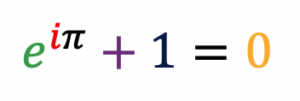# IB Maths

## Dr Luke is one of the best IB math tutorsDr Luke has been working as a IB math tutor  and providing one-to-one tutoring and student-organised group tutoring  since 1997.

With my extensive experience in scientific research and IB math tutoring, I can explain abstract concepts and theories by using tangible examples and plain language.  Learning needs intensive coaching just like learning to play tennis.  Lots of skills and theories can be grasped quickly through practices under individual coaching.  When one prepares a high-stakes test, the major challenge is short of time.  I believe that one-to-one tutoring, student-organised group tutoring, or small group tutoring are the most effective ways to get ready for the test. In these types of tutoring,  not only can test takers choose the topics they need to study and the pace with which they are comfortable, but I can tailor the materials to suit students’ background and needs. Students find that maths no longer abstract symbols, but fully embodying the reality. I stress to understand the logic developments and intrinsic connections of concepts and theories, which ultimately help students to understand subjects thoroughly.

Lots of students have gains confidence and skills in maths after they have learned with me for a period of time. I mainly help student to understand the concepts, formulae and theorems thoroughly and practise the basic skills. These help students to develop their mathematical gradually and to lay a solid foundation for their further studies. Reasoning in maths plays a critical role for the studies in other subjects, such as physics, chemistry, and biology.

Learning needs intensive coaching just like learning to play tennis. Lots of skills and theories can be grasped quickly through practices under individual coaching. As a IB math tutor, I believe that one-to-one tutoring, student-organised group tutoring, or small group tutoring are the most effective ways to get ready for the test. In these types of tutoring, not only can test takers choose the topics they need to study and the pace with which they are comfortable, but I can tailor the materials to suit students’ background and needs.

IB Mathematical studies standard level course includes 130 hours of instruction on eight topics. Students have 20 hours to spend project work – an individual piece of work.

• Introduction to the graphic  display calculator
Number and algebra
Sets, logic and probability
Functions
Geometry and trigonometry
Statistics
Introductory differential calculus
Financial mathematics

The IB Diploma Programme mathematics higher level course includes 190 hours of instruction on seven topics

• Algebra
Functions and equations
Circular functions and trigonometry
Matrices
Vectors
Statistics and probability
Calculus

Students also have spend 40 hours in one of the following topics

• Statistics and probability
Sets, relations and groups
Series and differential equations
Discrete mathematics

Students have 10 hours to develop a portfolio including two individual pieces of work based on mathematical investigation and mathematical modelling.